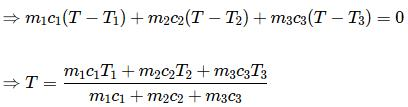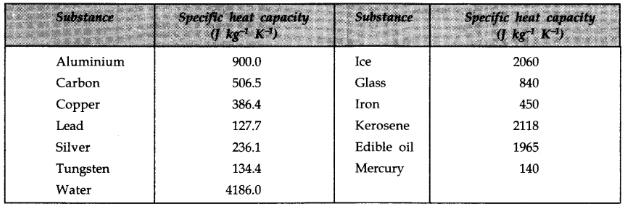Courses

# Test: Calorimetry

## 10 Questions MCQ Test Physics Class 11 | Test: Calorimetry

Description
This mock test of Test: Calorimetry for JEE helps you for every JEE entrance exam. This contains 10 Multiple Choice Questions for JEE Test: Calorimetry (mcq) to study with solutions a complete question bank. The solved questions answers in this Test: Calorimetry quiz give you a good mix of easy questions and tough questions. JEE students definitely take this Test: Calorimetry exercise for a better result in the exam. You can find other Test: Calorimetry extra questions, long questions & short questions for JEE on EduRev as well by searching above.
QUESTION: 1

### Water is used as coolant in automobiles radiators because

Solution:

Water is used as a coolant in automobiles radiators because it has high specific heat capacity. So, it absorbs a large amount of heat for a degree rise in temperature.

QUESTION: 2

### Equal masses of three liquids of specific heats C1, C2 and C3 at temperatures t1, t2 and t3 respectively are mixed. If there is no change of state, the temperature of the mixture is

Solution:

For the composite system, energy conservation yields no net energy flow in or out of the system.
Let final temperature be T
Then, heat absorbed by A+heat absorbed by B+heat absorbed by C=0Here in the question m1=m2=m3

QUESTION: 3

### Which of the given relation is true for molar heat capacity of a substance?

Solution:
QUESTION: 4

A piece of iron of mass 100g is kept inside a furnace for a long time and then put in a calorimeter of water equivalent 10g containing 240g of water at 20°C. The mixture attains an equilibrium temperature of 60°C. Find the temperature of the furnace. Specific heat capacity of iron = 470J/kg-°C.

Solution:

Mass of Iron = 100g
Water Eq of caloriemeter = 10g
Mass of water = 240g
Let the Temp. of surface = 0ºC
Siron = 470J/kg°C

Total heat gained = Total heat lost.

So,100/1000× 470 × (θ – 60) = 250/1000 × 4200 × (60 – 20)
⇒ 47θ – 47 × 60 = 25 × 42 × 40
⇒  θ = 4200 + 2820/47= 44820/47 =953.61°C

QUESTION: 5

A device in which heat measurement can be made is called

Solution:

A calorimeter is an object used for calorimetry, or the process of measuring the heat of chemical reactions or physical changes as well as heat capacity.

QUESTION: 6

Among the following substances, which one has highest specific heat capacity?

Solution:QUESTION: 7

The amount of heat required to raise the temperature of one mole of an ideal mono atomic gas through 2°C at constant pressure is (universal gas constant = R)

Solution:

At constant pressure
dQ= nCpdT
=1*(5R/2)*2
=5R

QUESTION: 8

5 g of ice at 0° C is mixed with 10 g of water at 10° C. The temperature of the mixture is:

Solution:

Heat absorbed by 5g ice when it converted to at 0° C = 5 x 80 = 400 cal.
Heat liberated by 10g water at 10° C to 0° C = 100 cal
Hence there is 15g water at 0° C and 300 cal needs to be liberated , thus for some amount of water converts into ice, hence the temp of mixture is 0° C.

QUESTION: 9

Which of the following relation is true for the specific heat capacity of substance?

Solution:

∆Q =mS∆T

QUESTION: 10

According to law of calorimetry, which of the given relation is true?

Solution:

A principle of calorimetry states that if there is no loss of heat in surrounding the total heat lost by hot body equal to the total heat gained by a cold body.
i.e. heat loss = heat gain